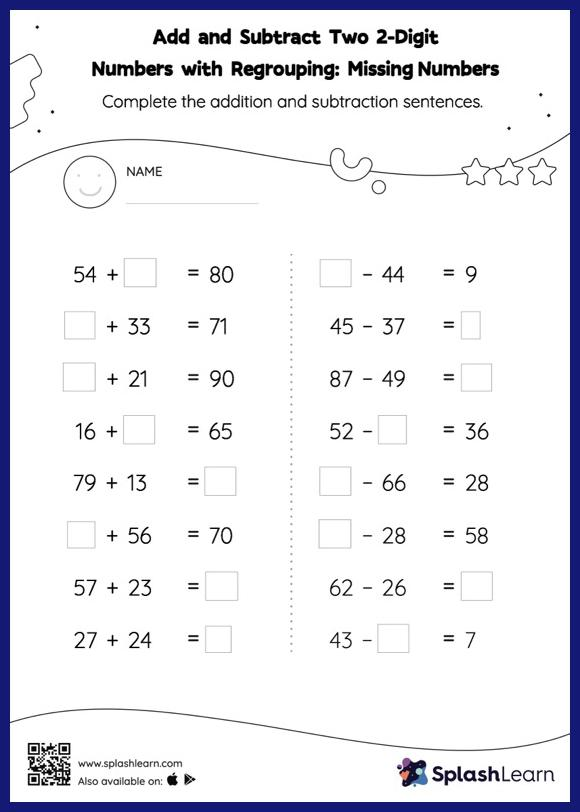# Add and Subtract Two 2-Digit Numbers with Regrouping: Missing Numbers Worksheet

Home > Add and Subtract Two 2-Digit Numbers with Regrouping: Missing NumbersChildren use their math skills to complete this add and subtract two 2-digit numbers with regrouping worksheet. Students use the relationship between addition and subtraction to find the missing number in this worksheet. They also need to regroup numbers to get to the answer in add and subtract two 2-digit numbers with regrouping worksheet. This worksheet is about practicing with the horizontal format in which numbers are written side by side. To develop flexibility with numbers and operations, students need to have enough practice in this format and not just rely on the vertical/column method.